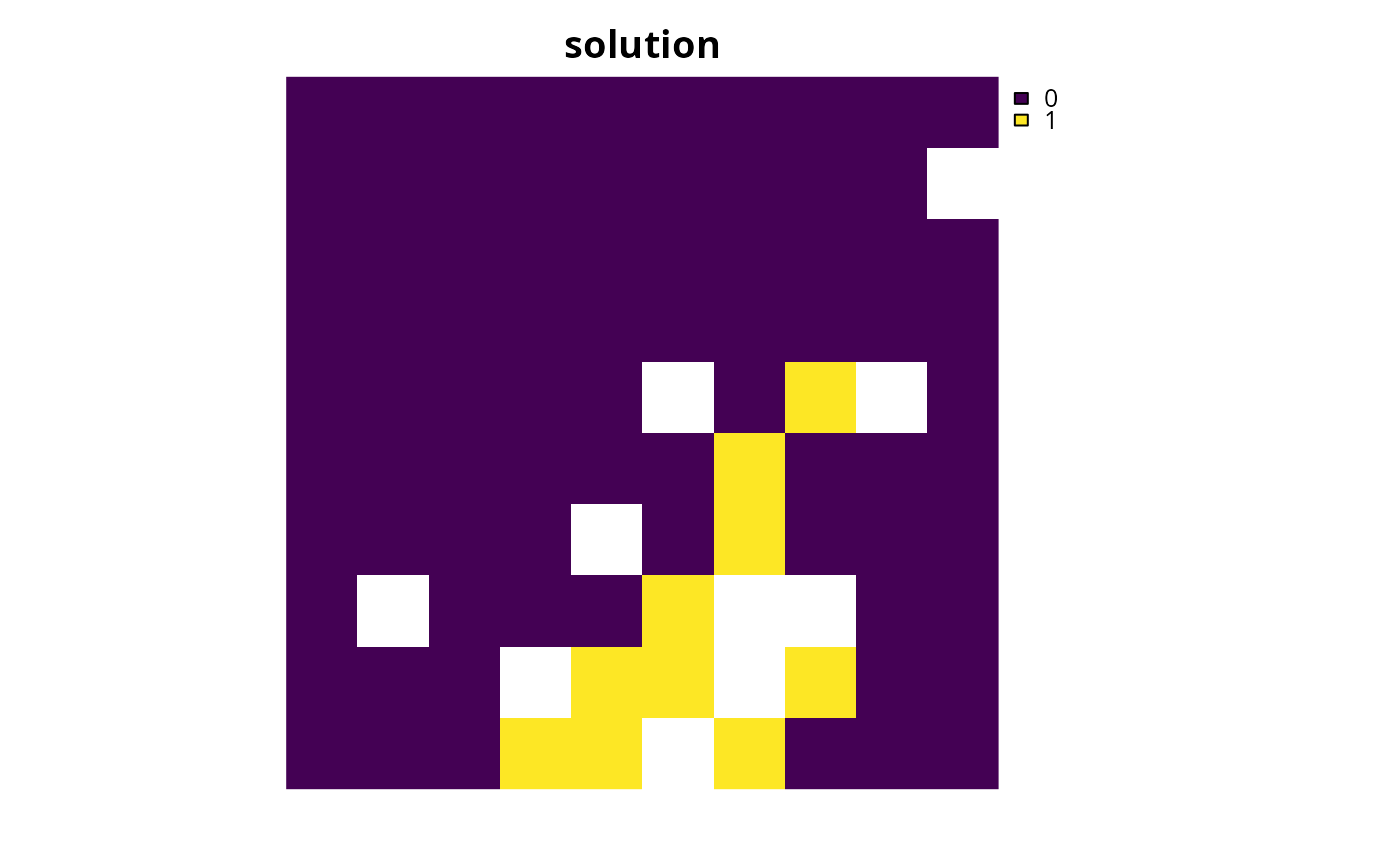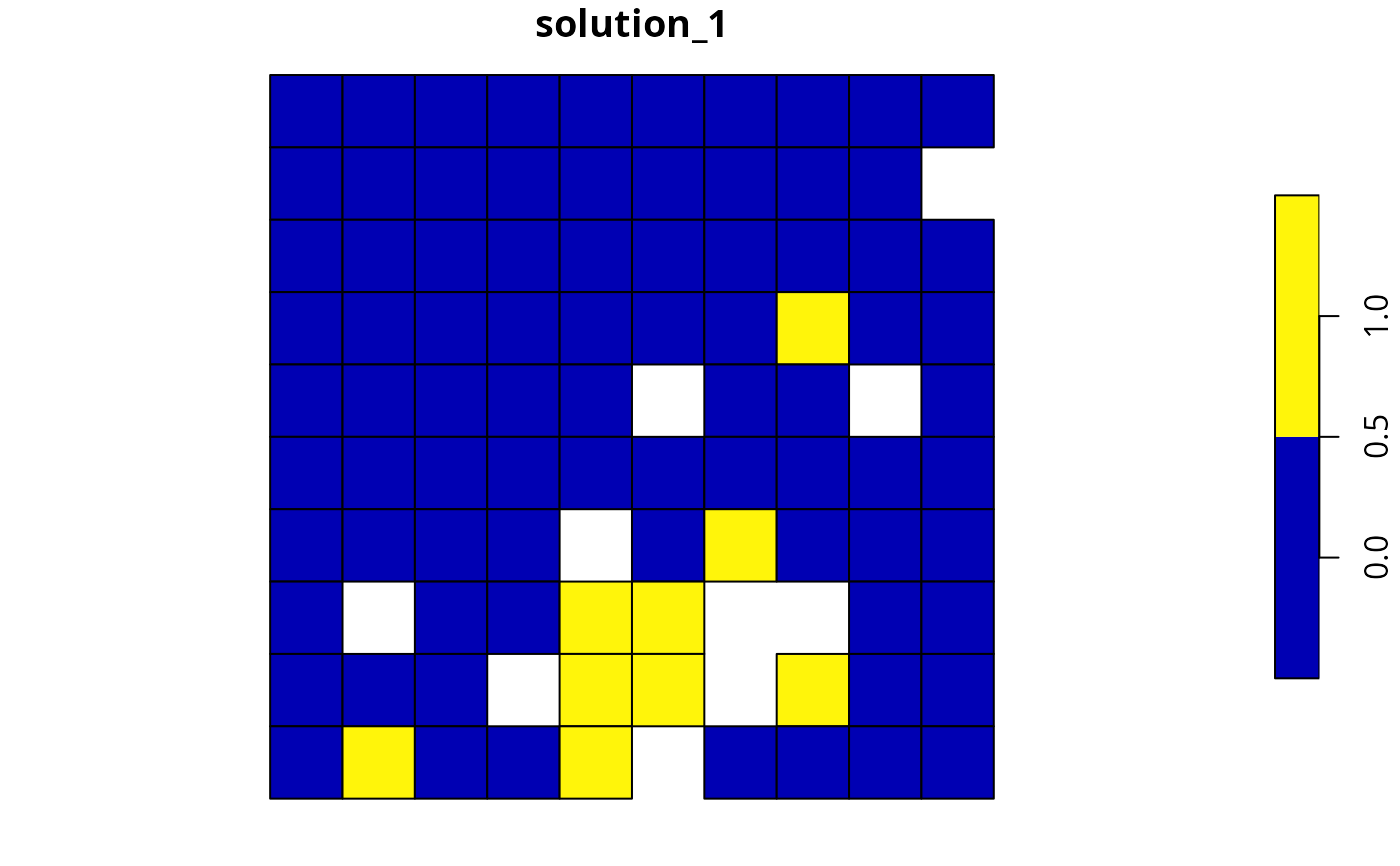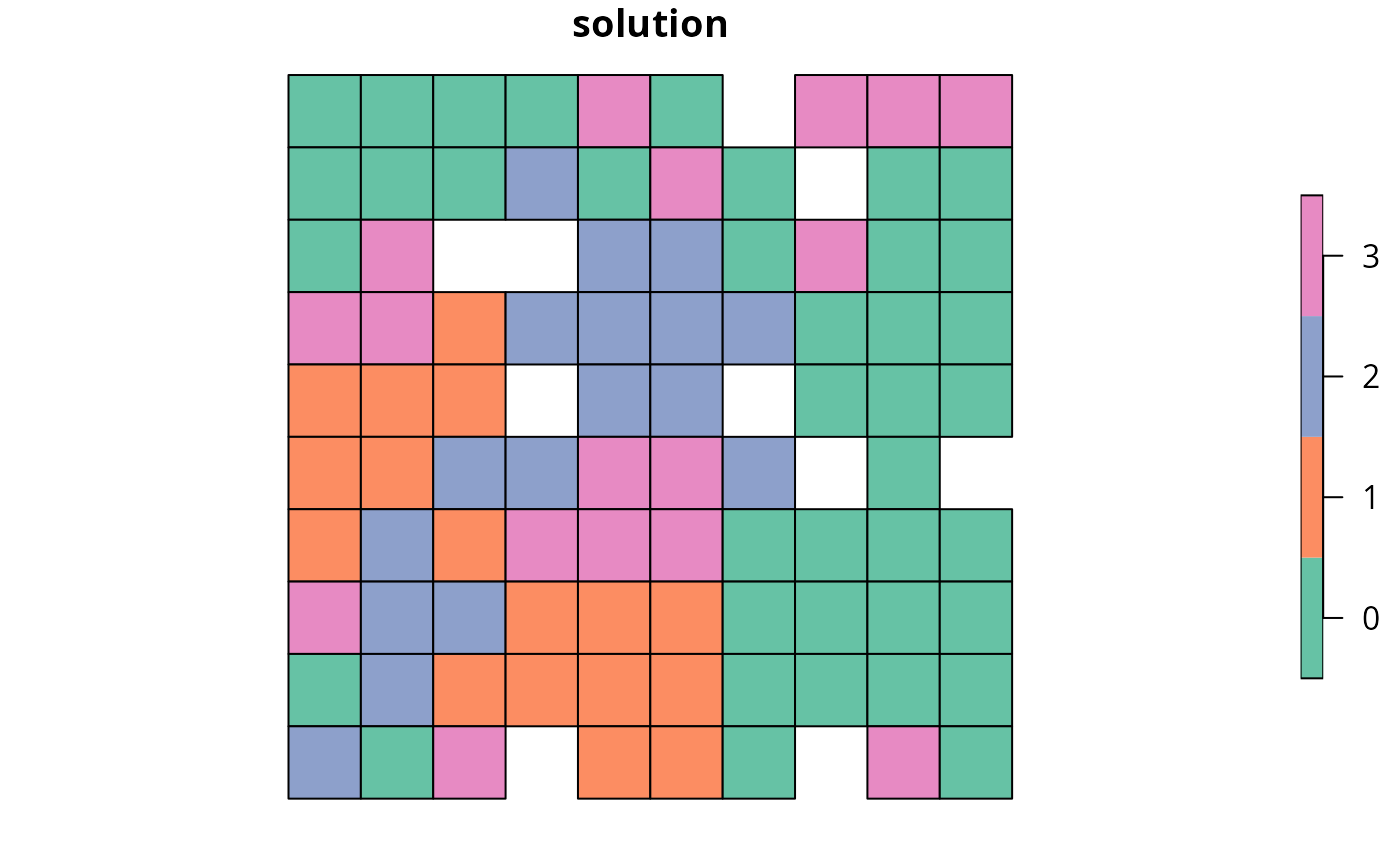Calculate the number of planning units selected within a solution to a conservation planning problem.

## Usage

eval_n_summary(x, solution)

## Arguments

x

problem() object.

solution

numeric, matrix, data.frame, terra::rast(), or sf::sf() object. The argument should be in the same format as the planning unit cost data in the argument to x. See the Solution format section for more information.

## Value

A tibble::tibble() object containing the number of planning units selected within a solution. It contains the following columns:

summary

character description of the summary statistic. The statistic associated with the "overall" value in this column is calculated using the entire solution (including all management zones if there are multiple zones). If multiple management zones are present, then summary statistics are also provided for each zone separately (indicated using zone names).

n

numeric number of selected planning units.

## Details

This summary statistic is calculated as the sum of the values in the solution. As a consequence, this metric can produce a non-integer value (e.g., 4.3) for solutions containing proportion values (e.g., generated by solving a problem() built using the add_proportion_decisions() function).

## Solution format

Broadly speaking, the argument to solution must be in the same format as the planning unit data in the argument to x. Further details on the correct format are listed separately for each of the different planning unit data formats:

x has numeric planning units

The argument to solution must be a numeric vector with each element corresponding to a different planning unit. It should have the same number of planning units as those in the argument to x. Additionally, any planning units missing cost (NA) values should also have missing (NA) values in the argument to solution.

x has matrix planning units

The argument to solution must be a matrix vector with each row corresponding to a different planning unit, and each column correspond to a different management zone. It should have the same number of planning units and zones as those in the argument to x. Additionally, any planning units missing cost (NA) values for a particular zone should also have a missing (NA) values in the argument to solution.

x has terra::rast() planning units

The argument to solution be a terra::rast() object where different grid cells (pixels) correspond to different planning units and layers correspond to a different management zones. It should have the same dimensionality (rows, columns, layers), resolution, extent, and coordinate reference system as the planning units in the argument to x. Additionally, any planning units missing cost (NA) values for a particular zone should also have missing (NA) values in the argument to solution.

x has data.frame planning units

The argument to solution must be a data.frame with each column corresponding to a different zone, each row corresponding to a different planning unit, and cell values corresponding to the solution value. This means that if a data.frame object containing the solution also contains additional columns, then these columns will need to be subsetted prior to using this function (see below for example with sf::sf() data). Additionally, any planning units missing cost (NA) values for a particular zone should also have missing (NA) values in the argument to solution.

x has sf::sf() planning units

The argument to solution must be a sf::sf() object with each column corresponding to a different zone, each row corresponding to a different planning unit, and cell values corresponding to the solution value. This means that if the sf::sf() object containing the solution also contains additional columns, then these columns will need to be subsetted prior to using this function (see below for example). Additionally, the argument to solution must also have the same coordinate reference system as the planning unit data. Furthermore, any planning units missing cost (NA) values for a particular zone should also have missing (NA) values in the argument to solution.

See summaries for an overview of all functions for summarizing solutions.

Other summaries: eval_asym_connectivity_summary(), eval_boundary_summary(), eval_connectivity_summary(), eval_cost_summary(), eval_feature_representation_summary(), eval_target_coverage_summary()

## Examples

# \dontrun{
# set seed for reproducibility
set.seed(500)

sim_pu_raster <- get_sim_pu_raster()
sim_pu_polygons <- get_sim_pu_polygons()
sim_features <- get_sim_features()
sim_zones_pu_polygons <- get_sim_zones_pu_polygons()
sim_zones_features <- get_sim_zones_features()

# build minimal conservation problem with raster data
p1 <-
problem(sim_pu_raster, sim_features) %>%

# solve the problem
s1 <- solve(p1)

# print solution
print(s1)
#> class       : SpatRaster
#> dimensions  : 10, 10, 1  (nrow, ncol, nlyr)
#> resolution  : 0.1, 0.1  (x, y)
#> extent      : 0, 1, 0, 1  (xmin, xmax, ymin, ymax)
#> coord. ref. : Undefined Cartesian SRS
#> source(s)   : memory
#> varname     : sim_pu_raster
#> name        : layer
#> min value   :     0
#> max value   :     1

# plot solution
plot(s1, main = "solution", axes = FALSE)# calculate number of selected planning units within solution
r1 <- eval_n_summary(p1, s1)
print(r1)
#> # A tibble: 1 × 2
#>   summary     n
#>   <chr>   <dbl>
#> 1 overall    10

# build minimal conservation problem with polygon data
p2 <-
problem(sim_pu_polygons, sim_features, cost_column = "cost") %>%

# solve the problem
s2 <- solve(p2)

# plot solution
plot(s2[, "solution_1"])# print solution
print(s2)
#> Simple feature collection with 90 features and 4 fields
#> Geometry type: POLYGON
#> Dimension:     XY
#> Bounding box:  xmin: 0 ymin: 0 xmax: 1 ymax: 1
#> Projected CRS: Undefined Cartesian SRS
#> # A tibble: 90 × 5
#>     cost locked_in locked_out solution_1                                    geom
#>    <dbl> <lgl>     <lgl>           <dbl>                           <POLYGON [m]>
#>  1  216. FALSE     FALSE               0     ((0 1, 0.1 1, 0.1 0.9, 0 0.9, 0 1))
#>  2  213. FALSE     FALSE               0 ((0.1 1, 0.2 1, 0.2 0.9, 0.1 0.9, 0.1 …
#>  3  207. FALSE     FALSE               0 ((0.2 1, 0.3 1, 0.3 0.9, 0.2 0.9, 0.2 …
#>  4  209. FALSE     TRUE                0 ((0.3 1, 0.4 1, 0.4 0.9, 0.3 0.9, 0.3 …
#>  5  214. FALSE     FALSE               0 ((0.4 1, 0.5 1, 0.5 0.9, 0.4 0.9, 0.4 …
#>  6  214. FALSE     FALSE               0 ((0.5 1, 0.6 1, 0.6 0.9, 0.5 0.9, 0.5 …
#>  7  210. FALSE     FALSE               0 ((0.6 1, 0.7 1, 0.7 0.9, 0.6 0.9, 0.6 …
#>  8  211. FALSE     TRUE                0 ((0.7 1, 0.8 1, 0.8 0.9, 0.7 0.9, 0.7 …
#>  9  210. FALSE     FALSE               0 ((0.8 1, 0.9 1, 0.9 0.9, 0.8 0.9, 0.8 …
#> 10  204. FALSE     FALSE               0   ((0.9 1, 1 1, 1 0.9, 0.9 0.9, 0.9 1))
#> # ℹ 80 more rows

# calculate number of selected planning units within solution
r2 <- eval_n_summary(p2, s2[, "solution_1"])
print(r2)
#> # A tibble: 1 × 2
#>   summary     n
#>   <chr>   <dbl>
#> 1 overall     9

# manually calculate number of selected planning units
r2_manual <- sum(s2$solution_1, na.rm = TRUE) print(r2_manual) #>  9 # build multi-zone conservation problem with polygon data p3 <- problem( sim_zones_pu_polygons, sim_zones_features, cost_column = c("cost_1", "cost_2", "cost_3") ) %>% add_min_set_objective() %>% add_relative_targets(matrix(runif(15, 0.1, 0.2), nrow = 5, ncol = 3)) %>% add_binary_decisions() %>% add_default_solver(verbose = FALSE) # solve the problem s3 <- solve(p3) # print solution print(s3) #> Simple feature collection with 90 features and 9 fields #> Geometry type: POLYGON #> Dimension: XY #> Bounding box: xmin: 0 ymin: 0 xmax: 1 ymax: 1 #> Projected CRS: Undefined Cartesian SRS #> # A tibble: 90 × 10 #> cost_1 cost_2 cost_3 locked_1 locked_2 locked_3 solution_1_zone_1 #> <dbl> <dbl> <dbl> <lgl> <lgl> <lgl> <dbl> #> 1 216. 183. 205. FALSE FALSE FALSE 0 #> 2 213. 189. 210. FALSE FALSE FALSE 0 #> 3 207. 194. 215. TRUE FALSE FALSE 0 #> 4 209. 198. 219. FALSE FALSE FALSE 0 #> 5 214. 200. 221. FALSE FALSE FALSE 0 #> 6 214. 203. 225. FALSE FALSE FALSE 0 #> 7 211. 209. 223. FALSE FALSE FALSE 0 #> 8 210. 212. 222. TRUE FALSE FALSE 0 #> 9 204. 218. 214. FALSE FALSE FALSE 0 #> 10 213. 183. 206. FALSE FALSE FALSE 0 #> # ℹ 80 more rows #> # ℹ 3 more variables: solution_1_zone_2 <dbl>, solution_1_zone_3 <dbl>, #> # geom <POLYGON [m]> # create new column representing the zone id that each planning unit # was allocated to in the solution s3$solution <- category_vector(
s3[, c("solution_1_zone_1", "solution_1_zone_2", "solution_1_zone_3")]
)
s3$solution <- factor(s3$solution)

# plot solution
plot(s3[, "solution"])# calculate number of selected planning units within solution
r3 <- eval_n_summary(
p3, s3[, c("solution_1_zone_1", "solution_1_zone_2", "solution_1_zone_3")]
)
print(r3)
#> # A tibble: 4 × 2
#>   summary     n
#>   <chr>   <dbl>
#> 1 overall    52
#> 2 zone_1     17
#> 3 zone_2     18
#> 4 zone_3     17
# }